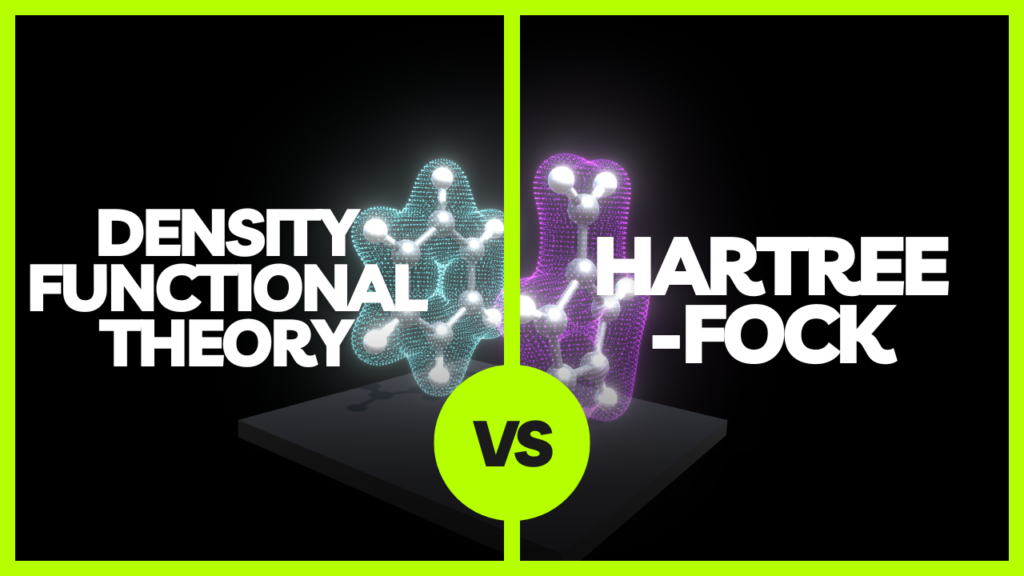# What is the difference between DFT and Hartree-Fock method?Density Functional Theory (DFT) and Hartree-Fock (HF) are both methods used in computational quantum chemistry to calculate the electronic structure of atoms and molecules. However, there are some key differences between the two methods.

1. One of the main differences between DFT and HF is the way that they treat electron correlation. In DFT, electron correlation is treated using an exchange-correlation functional, which is a mathematical approximation of the many-body effects of electron correlation. In contrast, HF does not include electron correlation (beyond that provided by the antisymmetry of the wave function), which makes it less accurate but computationally simpler (One needs to apply post-HF methods like MP2 to account for some of electron correlation). As a result, DFT is generally more accurate than HF for many types of calculations, particularly for systems with strong electron correlation.
2. Another key difference between DFT and HF is the way that they treat the exchange energy of the electrons. In DFT, the exchange energy is treated using the exchange-correlation functional, while in HF it is treated using the exchange operator, which is a mathematical representation of the way that electrons interact with each other. This difference in treatment can lead to differences in the calculated electronic structure of a system, and can affect the overall accuracy of the calculations.
3. Additionally, DFT only considers the electrons’ density, whereas HF considers the wavefunction of each electron individually. The functional in DFT depends on the density of the electrons, whereas the Hartree-Fock equations depend on the wavefunction of each electron.

Overall, while both DFT and HF are powerful methods for calculating the electronic structure of atoms and molecules, DFT is generally considered to be more accurate and versatile, and is therefore the more commonly used method for many types of calculations.

If I missed something or you find a mistake in the above article then please write to me in the comments down below.

### References

[wpedon id="7041" align="center"]

## 2 thoughts on “What is the difference between DFT and Hartree-Fock method?”

1. Very Good. A perfect text. Congratulation!!! But the HF method have not correlation, both dynamical and statical.

1. You’re 💯% correct. I have updated it now.

This site uses Akismet to reduce spam. Learn how your comment data is processed.